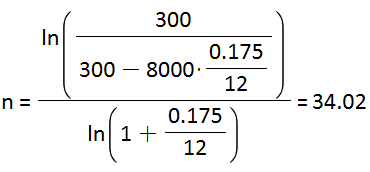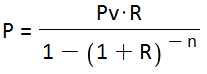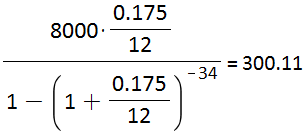# Credit Card Math

Running up a balance is easy, but paying it down is difficult. Your statement may suggest a minimum payment – but if you stick to this, you’ll be in debt for the long-haul.

You owe it to yourself to think about the math – it’s important! Knowing the equations gives you control – you can

• define a monthly payment and calculate how long it’ll take to pay down your debt
• or set your set a payoff date and calculate your monthly payments

## Credit Card Calculator

This Excel spreadsheet lets you experiment with payment amounts, APR’s and payoff times.## How Much Interest are you Paying?

Credit card interest is usually compounded daily. Here’s an easy way of approximating the interest portion

interest charge = average daily balance in one month x APR x number of days in month / 365

Assuming an average daily balance of \$8000 and an APR of 17.5%, the interest paid in month with 30 days is 8000 x 0.175 x 30 / 365 = \$115.07.

Bear in mind that this is an approximation. Working out the precise amount of interest requires calculating the interest on the balance each day during the month.

Let’s say you know your balance, your monthly payment and the annual interest rate. This equation gives the number of payments needed to reduce your balance to zero.

• n is the number of payments
• Pv is the credit card balance
• P is the periodic payment
• R is the interest rate

R is often defined as APR/12. If so, the number of payments n is in months.

Let’s say that your balance is \$8000, monthly payment is \$300 and the APR is 17.5%. Substituting these numbers into the equation givesSo the balance is paid off in just over 34 months.

This equation looks complicated, but is automated with Excel’s NPER function.

This equation can be rearranged to give the monthly payment in terms of the number of payments, interest rate and R. The equation isLet’s say that your balance is \$8000, APR is 17.5% and the number of monthly payments is 34. Substituting these numbers into the equation……gives you a monthly payment of \$300.11